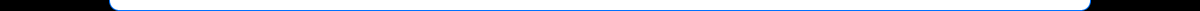HomeProblems inCurrent CosmologyTheoryOverviewChaptersSaturn RingsGalaxies/NebulaeMorphologyBlog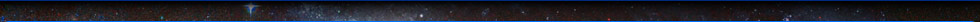At any given time, our knowledge of the natural world can be summarized by means of physical laws. These laws are used to construct mathematical models to describe essential aspects of an existing physical system. As we broaden our domain of observation, we must constantly verify whether these models, and their embedded laws, continue to be valid. Ideally, an existing model should be repeatedly tested and critiqued via an iterative process, in which new experiments are conducted and their results compared with the predictions of the mathematical model. A model that remains consistent with all measurements and observations will gain in status. In cases where its predictions deviate from the results of new experiments, a model must be adjusted to fit both the prior and the new sets of results. As science progresses and the number of iterations grows, an existing model may become increasingly difficult to adjust. Eventually a paradigm shift might offer radical simplification, and a new model may emerge to replace the old one. This process should be governed by the principle of Occams Razor, which states that the explanation of any phenomenon should make as few assumptions as possible. This principle can be expressed as, If all other things being equal, the simplest solution is the best. In accordance with Occams Razor, most successful models are initially developed with a relatively simple structure of few variables governed by few equations. However, in most cases there is a clear trade-off between the simplicity of a model and its accuracy. As new measurements deviate from the models predictions by an amount that cannot be discounted simply as measurement noise or measurement error, the model must be adjusted in order to fit reality. Inevitably, this iterative process increases the models complexity. Therefore, it is important not only to verify (after each iteration) that the modified model is consistent with the entire database of new and old experiments, but also to substantiate that the model is still consistent with Occams Razor, namely that the modified model is still the simplest model that explains the behavior of the given physical system. This second criterion, however, is often overlooked or lost in the process. One of the most fundamental and powerful models used in physics combines Newtons classical mechanics, which describes the relationship between force and the motion of bodies, and Newtons law of universal gravitation. Newton based his theory on three simple postulates and two simple equations that have since been demonstrated to provide an efficient and astonishingly accurate model of most ordinary mechanical situations. Over 200 years of scientific advances transpired before measurements started to suggest the need for a departure from the Newtonian model. Newtons law of universal gravitation and his laws of motion were based on prior work done by Galileo and Kepler. Johannes Kepler, a German mathematician, studied observations of planetary dynamics tabulated by the Danish astronomer Tycho Brahe and developed an ad hoc mathematical model to describe the empirical observations via three laws of planetary motion.1 Keplers laws provided a clear blueprint with which an astronomer of the time could calculate and predict the location and the periodicity of planets in the Solar System. While his achievement was incredibly important, Keplers mathematical laws were empirical in nature, and were not derived from any basic underlying principles. The rationale and explanation of the theoretical principles driving these rules emerged over half a century later with Newtons publication of his Philosophiae Naturalis Pricipia Mathematica. As Newtons mathematical model was based on a more profound understanding of fundamental principles, the shortcomings of the Keplerian model became apparent. Keplers laws were found to be inaccurate when the mass of an orbiting object is not negligible in comparison to the mass of the object being orbited. Furthermore, Keplers laws did not deal with situations in which the smaller body is moving faster than the escape velocity, as in the case of comets. Under such circumstances, the small body trajectory becomes an open parabolic or hyperbolic orbit, rather than a closed elliptical orbit. In addition, Keplers laws assumed a two-body system and could not be applied accurately to systems containing three or more bodies.2 Newtons insight therefore provided a simpler and more accurate way of calculating planetary trajectories, which not only resolved the limitations of Keplerian dynamics, but also substantially increased the scope of applications covered by the model. The basic framework for Newtons theory was also inspired by the work of Italian astronomer Galileo Galilei, introduced in 1638. Before Galileo, ideas about the motion of bodies were based on Aristotles assertion that the natural state of a body was to be at rest and that a resting body will move only if driven by a force or impulse. Aristotle further intuited that a heavy body should fall faster than a lighter one, as the heavy body should by nature experience greater pull toward the earth. Galileo put these notions to a test. By rolling balls of different weights down a smooth slope, Galileo created an effect similar to bodies falling vertically, and found that contrary to Aristotles predictions, the speed of all bodies increased at the same rate regardless of their mass (as long as air resistance and friction can be neglected). In other words, the gravitational acceleration of an object is independent of its mass. This idea led Einstein, almost three centuries later, to his equivalence principle and to the development of the general theory of relativity. Galileos experiment further demonstrated that the effect of a force is to change the speed of a body, rather than simply to set it in motion. It followed that a body moving on a level surface will continue to travel in the same direction at constant speed unless disturbed, a principle that became Newtons first law. Galileo also formed the principle of Galilean relativity, stating that dynamical laws are precisely the same when referred to in any non-accelerating frame of reference, where reference frames travel at constant velocity relative to each other. Hence, there is no experiment that can differentiate between a system at rest and a system in uniform motion, and there is no absolute motion or absolute rest state. This principle initially provided the basic framework for Newtons theory and later on inspired Einsteins special theory of relativity. Newtons original formulation of his laws of motion stated the principle of Galilean relativity as one of five or six fundamental laws. Later on, however, Newton adopted the notion of absolute space and simplified his model to rely on only three independent laws: Newtons First Law: A particle will stay at rest or continue to move at a constant velocity following a straight line unless acted upon by an external unbalanced force. Newtons Second Law: Observed from an inertial frame (a frame at rest or moving at constant velocity) the net force on a particle is equal to the particle mass times its acceleration, or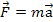. Newtons Third Law: Whenever a particle A exerts force on a second particle B, B simultaneously exerts a force of same magnitude in the opposite direction on particle A. Newton then formulated a law to describe the gravitational force, stating that two point objects of mass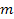and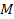attract each other along the line that connects them via a force that is proportional to the product of their masses divided by the square of the distancethat separates them. Expressed mathematically, Equation 1-1whereis the gravitational vector force applied on either point particle, G is a gravitational constant, andis the unit vector in the direction toward the other point object. Newtons gravitational force thus describes a central force, which can also be stated as a conserving force that is derived from a potential energy functionvia Equation 1-2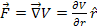, where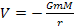Remarkably, just a few simple rules result in an extraordinarily powerful and versatile theory that predicts the behaviors of macroscopic objects with great accuracy, as long as their speeds are non-relativistic. For his theory to work Newton had to assume, against his own judgment and instincts, that gravity is instantaneous, or in other words that the speed of gravity is infinite. Newton was also quite uncomfortable with the notion implied by his equations that gravity is an action at a distance, without direct contact between the bodies involved. While no mathematical model can exactly reproduce an actual physical system, the Newtonian theory remained virtually unchallenged for over 200 years. That is, until Albert Einstein introduced his revolutionary special theory of relativity, which provided a more accurate model in the case of object velocities that approach the speed of light, and his new gravitational theory, the general theory of relativity, which resolved a number of shortcomings within Newtons theory of gravitation. Special relativity, and to a larger extent general relativity, significantly increased the mathematical complexity of both mechanical dynamics and gravitation, resulting in a set of non-linear equations that often require resolution via computer-based simulations. However, both theories are complete and self-consistent theories based on strikingly simple postulates. Special relativity relies on the principle of relativity and also postulates that the speed of light is the same in all inertial frames. General relativity relies on the principles of special relativity and on the equivalence principle.3 Both theories converge to the Newtonian gravitational model in the limiting case of slow velocities (in the case of special relativity) or weak gravitational fields (in the case of general relativity), where their equations approach Newtons equations. Due to the minimalistic nature of their assumptions, special relativity and general relativity can be considered to comply with the spirit of Occams Razor, regardless of their substantial computational complexities. However, the simplicity of Newtons model, and the fact that the majority of known systems are non-relativistic, make it the preferred choice for most applications. Therefore, in spite of the somewhat reduced accuracy of the Newtonian model relative to general relativity or special relativity, it is still the most frequently used model in earthbound engineering applications, as well as in most areas of space exploration. As our domain of observation has broadened considerably in the recent century, studies have begun to show significant inconsistencies between observations and the predictions of both, Newtons theory and general relativity. Some of the new discoveries were attributed to non-gravitational phenomena, as in the case of the strong interaction between nucleons, which was classified as an entirely new force unrelated to gravitation and became part of the standard model. Other inconsistencies clearly related to gravitation were reconciled by modification of the gravitational model of the Universe in an iterative process, resulting in a significant increase in the complexity of the overall gravitational model. Ideally, every iteration step should have been tested to uphold the following criteria: 1. Can the modified model explain, quantify and be consistent with all prior known phenomena, as well as the new discoveries? 2. Is the modified model still the simplest one available? Or alternatively, can it be replaced by an equally successful, yet substantially simpler model? The risk in the failure to uphold the second criterion is that even a flawed model that agrees with all prior known phenomena can be modified to agree with new contradicting data by simply increasing the number of independent variables used, thus creating a more complex and still flawed model. The best way to eliminate flawed models, but by no account the easiest route to take, is by searching and testing for simpler alternative models. The current astronomical model of the universe, which describes the motion of a system of gravitating bodies, is based on the general theory of relativity (and therefore indirectly on the less complete Newtonian theory). The amount of visible matter observed in galaxies and galactic clusters is insufficient to explain the motion of stars in galaxies, or the motion of galaxies within clusters. We can therefore conclude that either the current understanding of gravitation is incorrect, or additional non-visible matter must exist. At present, most astrophysicists prefer the latter explanation, and consequently a new variable of dark matter has been added to the current paradigm. The addition of the dark matter concept made possible the formation of a plausible model that is consistent with observations without shaking the theoretical foundation of the established gravitational model. Since undetected, dark matter is believed to consist of non-baryonic matter and to interact through the gravitational force. To fit observations, dark matter must account for about 90% of the average galaxy mass, and furthermore must dominate the mass of the galaxy up to several hundred kiloparsecs from its center. However, no trace of dark matter has ever been observed directly and no effects of such matter can be measured within our own Solar System. The complexity of the dark matter model, and the lack of direct observational evidence, should raise the question of whether modification of the cosmological model with the inclusion of dark matter still provides the simplest possible model to explain the observed properties of galaxies as well as stellar and galactic motions, or whether a simpler model exists. Additional variables have been incorporated into the current accepted cosmological model in a series of iterations geared to resolve paradoxes related mainly to the Big Bang theory. The added variables include the assumption of the existence of dark energy, which together with dark matter brings the total amount of non-visible and undetected matter in the universe to as large as 96%, as well as a theory of inflation, which indicates that at some initial period of time, space expanded at a rate much higher than the speed of light. Newton never published the reasoning that guided him to his equation of universal gravitation, and we can only speculate the process by which he arrived at this final form, however, history provides us with a clear sense of the information available to Newton at the time. On no account could he have known about the phenomenon of constant rotation curves in spiral galaxies, where stars travel around the galactic center with roughly constant rotational speed, rather than at a speed decreasing inversely to the square root of the stars radius of orbit. While Galileo had observed a system of rings around Saturn prior to Newtons work, its characteristics were not yet understood. The difficulties of fully explaining planetary rings within the Newtonian theory have since proved to be formidable, as attested by the fact that almost 400 years later we are still very far from understanding the root of their existence. Newton did not have access to deep inelastic scattering experiments that have since brought to light the behavior of particles on a subatomic scale and could not have known about the existence of the short-range strong forces between nucleons within the nucleus. Such forces were later observed to be strong enough to overcome the Coulomb rejection force between protons, and therefore must be greater than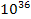times the strength of the gravitational force predicted by Newtons formula, at nuclear distances of the order of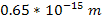. Although the strong force is considered as a separate force, entirely distinct from gravitation, it appears to share some of gravitations essential defining qualities. Similar to the gravitational force, for instance, the subatomic force appears to be (at least approximately) a central force between a pair of point-like particles with strong dependency on the respective masses of the interacting particles.4 An interesting yet speculative question is whether Newton would have attempted to integrate the additional observed phenomena into his theory of gravitation had the above information been available to him? We can only suppose Newtons course of action, but the question that should be addressed and may be contemplated is whether such phenomena, from long-range force interactions to force interactions on a nuclear level, can be explained by a single equation that provides a simpler and more accurate model than the current paradigm. In fact, there is a whole family of equations that, given the limited observation technology available to Newton and for about 230 years thereafter, would have provided virtually indistinguishable results and predictions from those developed by Newton at the measured distance ranges available at the time. However, on extremely small scales, and in some cases on galactic or cosmic scale distances, the predictions formulated by these equations may differ from each other, and from the predictions of the Newtonian equation. These distinctions could not have been addressed during Newtons time and would have been irrelevant to his reasoning, since the alternative equations are slightly more complex and would have been discounted in accordance with Occams Razor. If a simpler model does exist, it is likely to be based on a modified gravitational equation. To explore this notion, let us first analyze some simple alternative equations for the gravitational potential energy, starting with the exponential energy equation, Equation 1-3as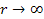Note thatis a constant with a dimension of distance. For reasons that will become apparent in the next chapter, a good choice forcould be in the vicinity of. This will result in the following force equation: Equation 1-4asThe choice made in equation 1-3 may seem odd and non-intuitive. However, equation 1-3 is practically indistinguishable from Newtons equation of potential energy (1-2) at the distance range of. Similarly, at these distances, the results of equation 1-4 cannot be distinguished from those of Newtons equation of force (1-1). Although the force in equation 1-4 is exponential, rather than proportional to, the difference between the force calculated via equation 1-4 and the Newtonian force calculated via equation 1-1 merely amounts to about 0.1% at a distance of, which is the Bohr radius, or roughly the radius of the hydrogen atom. At a distance of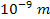the difference reduces to about 0.0057%, and at distances above one micrometer (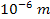), there is virtually no difference between the two calculated forces. In other words, if Newton had used the slightly more complex equation 1-4 (or 1-3) rather than equation 1-1 (or 1-2), he would have obtained the exact same results. Nevertheless, had the thought of using an exponential function occurred to Newton, the inability at the time to experimentally differentiate between the two sets of equations would have most likely steered him toward the simpler equation 1-1, in accordance with Occams Razor. While equations 1-3 and 1-4 are also quite simple, they contain an additional universal constantand require the calculation of an exponential function, which was not an easy task in the pre-calculator era of the 17th century. Today data is available on interactions at distances at which the predictions of Newtons equations of force and potential energy differ from those of equations 1-4 and 1-3, and the results of the two sets of equations are no longer identical within all measurement ranges. Had Newton selected equation 1-3 (or 1-4) as his law of gravitation, then three centuries later the discovery of a strong nuclear force may have possibly been perceived as an additional triumph for the theory of gravitation. After all, the newly discovered strong force was found to be approximately a central force5 between a pair of particles that is mainly dependent on the particle masses, and to demonstrate explosive growth at the distances, just as predicted by equations 1-3 and 1-4 due to the explosive growth of the exponential term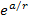at distances. The finding that a single mathematical equation may display behaviors that resemble at least some key characteristics of the strong force at extremely short distance ranges, while converging with Newtons gravitational equation at larger distances, does not necessarily imply that the two forces are one and the same. However, such a theory does not appear to have ever been considered. Instead, the strong force became a part of an extensive new theory called the standard model.6 Based on Newtons law of gravitation, the standard model was developed with the underlying assumption that the gravitational force is negligible at atomic and sub-atomic distances. Exponential equations such as 1-3 or 1-4 are not the only possible alternatives to Newtons gravitational equations 1-1 and 1-2. Of interest are also equations of the type Equation 1-5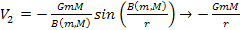as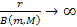and Equation 1-6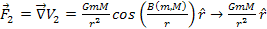aswhereis either a constant or a function of the particle masses. Equations 1-5 and 1-6 provide a perfect Newtonian attractive force at distances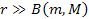, where they become virtually indistinguishable from the Newtonian equations. At shorter distances of, the potential energy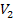begins to oscillate between a series of constant maxima and minima (andrespectively), at which point the force can also become repulsive. The cyclical nature of these equations may be functional in addressing the cause of the ring and spiral structures demonstrated in galaxies and planets, as well as for the shell structure and resonances of the atoms nucleus. However, in this form, these equations are unlikely to be consistent with the strong force, since it is not possible to find a function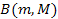that can simultaneously provide the high amplitude required at short distance ranges and the abrupt and explosive growth of the force at the distance range of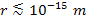. As it turns out, neither equation 1-3 nor equation 1-5 can reproduce and explain the entire range of observational data. However, merging these two concepts will be shown to provide a simpler and more accurate theory than the current paradigm, with the ability to explain a large number of yet unexplained phenomena. The hypothesis presented here, entitled the theory of unified gravitation (UG), holds that the nuclear strong interaction and gravitation are actually one and the same force, viewed at different distance scales. This force is predominantly a central force between a pair of fundamental particles,7 and can be derived from a central potential given by Equation 1-7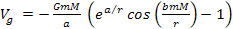The equation uses the gravitational constant , as well as two additional universal constants,and. Variablesand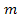represent the respective masses of two particles, andis the distance between the pair of particles. When applied to objects consisting exclusively of ordinary particles at distances significantly larger than 10 micrometers, the UG force equations developed in the first two chapters will be shown to yield results indistinguishable from those derived by Newtonian calculations within the experimental level of reliability.8 However, when applied to much heavier particles, the results obtained by the UG and Newtonian equations may diverge at much larger distance ranges, and the UG potential and its derived force are able to elucidate many thus far unexplained observations in the cosmic, galactic, planetary and nuclear realms. The ability of larger particles to form is demonstrated by high-energy collisions between electrons and positrons, which have been shown to produce particles that are more than 350,000 times their mass. Therefore, a secondary, but essential assumption of the UG theory holds that the high pressure and temperature conditions at the cores of large satellites, planets, stars, and galaxies provide the energy and means necessary to produce superheavy particles (SHPs) and to prevent their decay. The present book is intended to introduce the theory of Unified Gravitation (UG). It would be unreasonable to expect that the capability of any new theory to provide better agreement in one particular area is sufficient to challenge a well-established fundamental theory that for centuries provided a clear picture of the known universe. Therefore, the UG theory must be applied successfully to the analysis of a wide range of phenomena in multiple areas of physics. The purpose of this book, therefore, is to demonstrate sufficient evidence of the viability and the advantages of unified gravitation, in order to challenge and motivate the physics community toward further testing. The first three chapters are dedicated to developing the UG postulates and gravitational equations. The theory will subsequently be applied on solar, galactic and cosmological scales to explain a broad selection of observed phenomena. In the fourth and fifth chapters, a simple UG-based model will be demonstrated to provide for a number of the observed morphologies of astronomical systems. In Chapter IV, a simple model will be employed to reproduce a variety of complex morphologies observed in planetary nebulae and galaxies, from bipolar structures in figure 4-4 to ring structures in figure 4-5, to simple spiral structures in figure 4-8 to flocculent spirals in figure 4-9.9Figures 4-4d and 4-4e: A comparison between a bipolar structure calculated by the UG equations (figure 4-4d) and MyCn 18, the hourglass nebula (figure 4-4e). Image credit for figure 4-4e: R. Sahai and J. Trauger, Photo AURA/STScl/NASA/JPL (NASA photo # STScl-PRC96-07) http://apod.nasa.gov/apod/ap020615.html.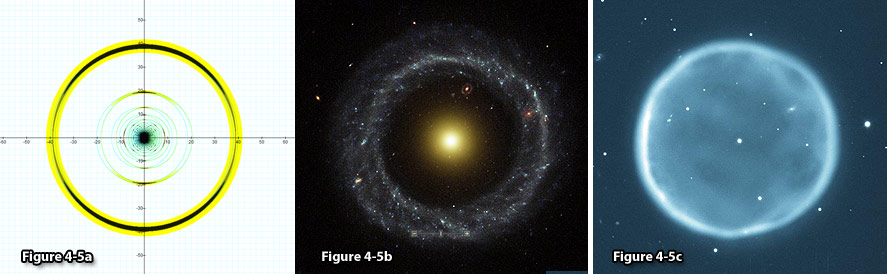Figure 4-5a: A UG calculated image of a ring morphology (on the left), compared with Hoags object and the planetary nebula Abell 39. Figure 4-5b: Hoags Object, image credit: NASA and the Hubble Heritage Team (STScI/AURA) Acknowledgment: R. Lucas(STScI/AURA) http://www.nasaimages.org/luna/servlet/detail/NVA2~4~4~4753~105279:Hoag-s-Object--A-Strange-Ring-Galaxy. Figure 4-5c: The Planetary Nebula Abell 39, WIYN Observatorys 3.5-m (138-inch) telescope at Kitt Peak National Observatory, Tucson, AZ. Image credit: NOAO/AURA/NSF.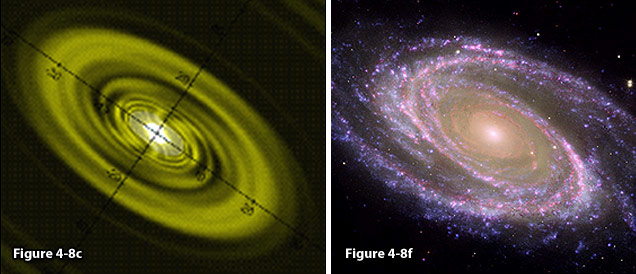Figure 4-8e: A modeled galaxy compared with the observed spiral galaxy M81 (figure 4-8f). Figure 4-8f: M81 image credit: NASAs Spitzer and Hubble space telescopes and NASAs Galaxy Evolution (NASA/JPL-Caltech/ESA /Harvard-Smithsonian CfA), http://www.nasa.gov/mission_pages/spitzer/multimedia/spitzer-20070604.html.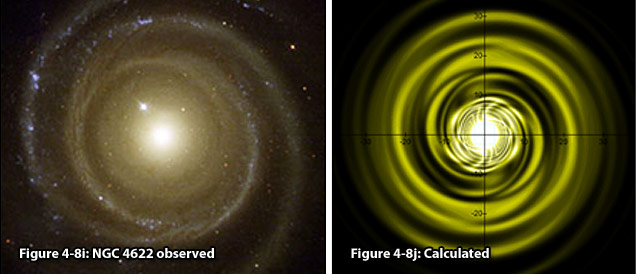Figure 4-8i: NGC 4622, image credit: G. Byrd, R. Buta, (Univ.Alabama, Tuscaloosa), T. Freeman (Bevill State College), NASA http://apod.nasa.gov/apod/ap040221.html. Figure 4-8j:A modeled spiral galaxy compared with the observed galaxy NGC 4622 (figure 4-8i), which displays both trailing and leading spiral arms.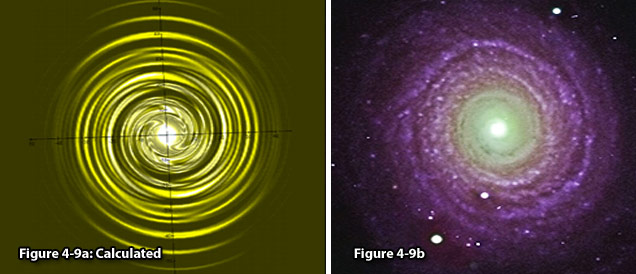Figure 4-9a:A modeled spiral galaxy compared with the observed galaxy NGC 488 (figure 4-9b), demonstrating a flocculent structure. Figure 4-9b:NGC 488, image credit: Johan Knapen and Nik Szymanek, Isaac Newton group of telescopes; http://www.ing.iac.es/PR/science/ngc488.html.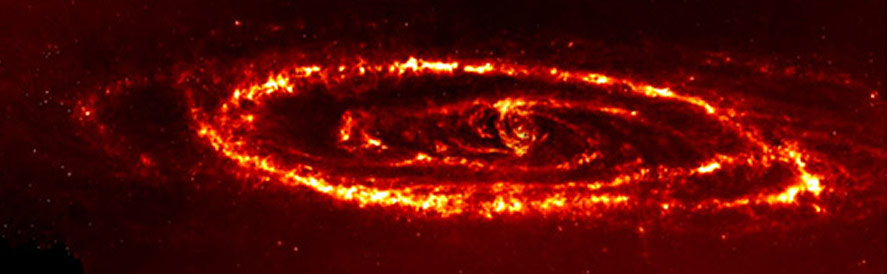Figure 4-7c: An infrared view of the Andromeda Galaxy taken by NASAs Spitzer Space Telescope. Image credit: NASA/JPL-Caltech/K. Gordon (University of Arizona), http://www.spitzer.caltech.edu/Media/releases/ssc2005-20/ssc2005-20a.shtml.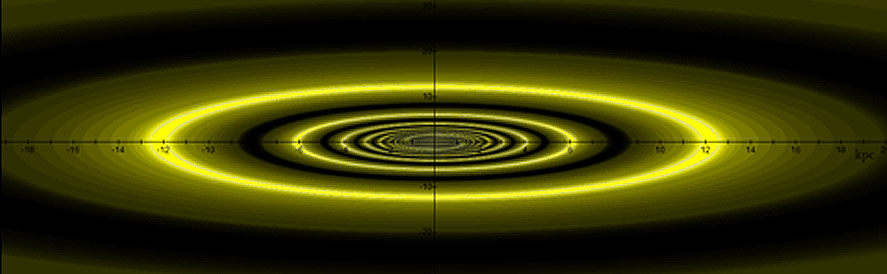Figure 4-7d: A UG calculated image of the Andromeda Galaxy.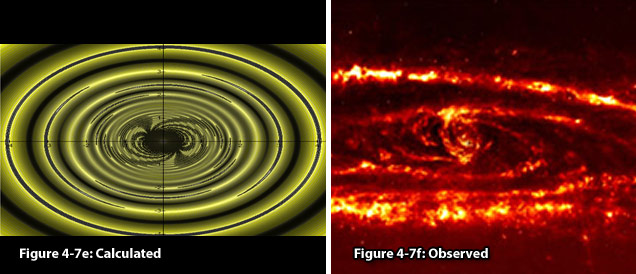Figure 4-7e: Calculated Figure 4-7f: Observed In figures 4-7d and e, a UG modeled galaxy is demonstrated to bear a resemblance to the general morphology and properties of the Andromeda galaxy. Note that the rotating distorted hourglass structure at the center of the calculated galaxy (figure 4-7e) bears a resemblance to the general structure of Andromedas nucleus (figure 4-7f), additionally accounting for the two short spirals observed to extend from the nucleus and for the ansae reported by Beaton et al. in 2005 (Beaton et al., 2005).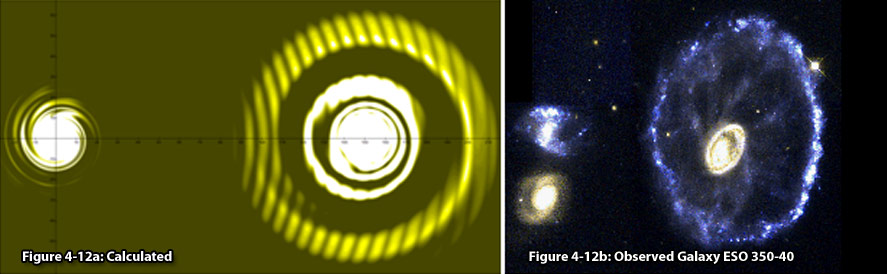Figure 4-12a: Depicts an image of the effect of a companion galaxy on a modeled galaxy, compared with the observed cartwheel galaxy ESO 350-40 (figure 4-12b). Note that both galaxies demonstrate clumps of star formation. Figure 4-12b: The Cartwheel Galaxy, ESO 350-40. Image credit: NASA, ESA, and K. Borne (STScI), http://hubblesite.org/gallery/album/pr2007017d. In Chapter V, the UG theory is applied to model the general structure of Saturns main body of rings. Saturns ring system was first observed by Galileo nearly 400 years ago, 77 years prior to Newtons discovery of the gravitational force. Despite the extensive study of planetary rings, the origin and many of the properties of Saturns complex ring system remain a mystery. Figure 5-1 provides a comparison between a UG calculated model and a computer-generated image of Saturns ring system using RSS 3-wavelength occultation data.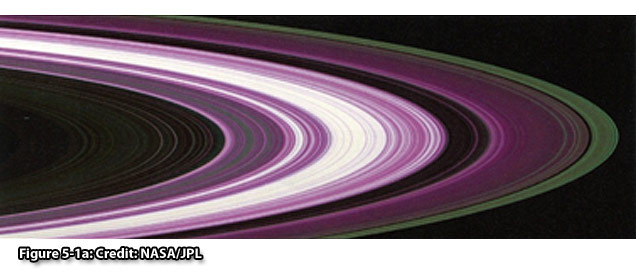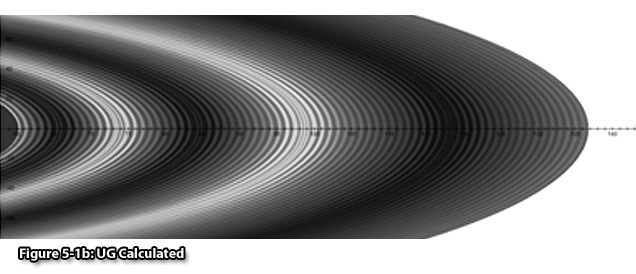A detailed, high resolution image of Saturns D ring is displayed in Figure 5-2. Figure 5-7a displays a detail of Saturns A ring interior to the Encke Gap taken by the Cassini ISS instrument. On the right the image reveals spiral bending waves, where the wavelength decreases inwards, and on the left a succession of spiral density waves, where the wavelength decreases outwards. The same structure is revealed in the calculated image 5-7c.Figure 5-2a:Saturns D ring, NASA/JPL/Space Science Institute, http://photojournal.jpl.nasa.gov/catalog/?IDNumber=PIA08990. Figure 5-2c:A comparison between a calculated image of Saturns D ring (figure 5-2c) and the observed D ring (figure 5-2a).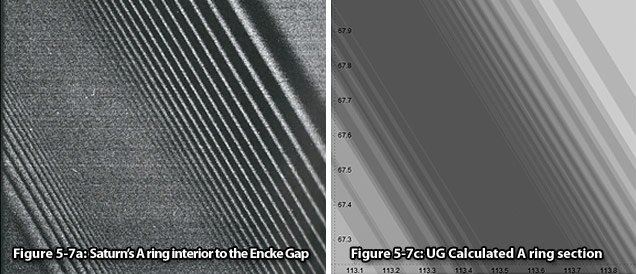Figure 5-7a: Saturns A ring interior to the Encke Gap. Image credit: NASA/JPL/Space Science Institute; http://photojournal.jpl.nasa.gov/catalogue/PIA06093. Building on the same equations, the UG theory will be applied in Chapter VI to explain galactic dynamics. The unified gravitational equations will be shown to provide for the constant velocity curve observed in spiral galaxies, as well as for high stellar velocities, without relying on the auxiliary hypothesis of dark matter. The chapter will additionally discuss the origin of galactic wind and the mechanism underlying star formation and fragmentation. Chapter VII will discuss the implications of unified gravitation on general relativity and on the current cosmological model. In addition, the formation of galactic voids, the triggering mechanism and the driving forces behind the massive outflow of gas and matter observed during novae or supernovae, the clustering of nearby galaxies, and the effective repulsion between distant galaxies will be addressed. Finally, the theory of unified gravitation will be demonstrated to provide an explanation for the observed expansion of the universe while avoiding the dilemmas that led to the adaptation of inflation theory and to the assumptions of dark matter and dark energy, thus providing a significantly simpler model. Additional applications of the UG equation will be discussed in the following chapters. Chapter VIII is concerned with the processes of ionization and their sources of energy. Chapter IX will discuss the structure and composition of planets and the mechanisms that determine whether a given planet will develop into a terrestrial or gas planet, and whether or not a given planet will demonstrate a ring system. The chapter will additionally propose a possible explanation for the generation of planetary magnetic fields. In Chapter X the question of galactic redshift periodicity will be contemplated. As the fundamental premise of the UG theory is the unification of gravitation and the strong interaction, the final chapter will demonstrate the applicability of the unified gravitational equations on nuclear scales, where the UG theory will be demonstrated to account for the observed weak fall-off of the deep inelastic scattering cross section, and to provide a scaling behavior similar to Bjorken scaling.     1 Keplers first law: the orbit of a planet around a star is an ellipse, with the star located at one of the foci. Keplers second law: identified that the line joining a planet and its star sweeps out equal areas during equal intervals of time. Keplers third law: the square of the sidereal period of an orbiting planet is directly proportional to the cube of the orbits semi-major axis, or2 Keplers laws are inadequate, for example, for calculating the orbit of the Moon, as the calculation requires taking into account three heavenly bodies, the Sun, the Earth and the Moon. 3 The equivalence principle will be discussed later in this chapter. 4 To be further discussed in Chapter II. 5 As matter of fact, the strong force was shown to deviate slightly from being a central force. This will be explained to be a relativistic effect, similar to the one that makes central Coulomb force appear as a non-central magnetic force when viewed from an inertial frame moving at high velocity relative to the charges. 6 Interestingly, one of the declared goals of the standard model is to eventually unify the four fundamental forces of nature; the electromagnetic force, the strong interaction, the weak interaction and the gravitational force. 7 Fundamental particles include any barion, mason or lepton, while ordinary matter refers to any matter constructed exclusively from protons, neutrons and/or electrons. 8 The experimental margin of error is derived from the published variability of the gravitational constant . 9 Note that the figures are numbered according to their numbering in the chapters in which they are presented.